# Simple layers

Simple Modules are used for various tasks like adapting Tensor methods and providing affine transformations :

• Parameterized Modules :
• Linear : a linear transformation ;
• SparseLinear : a linear transformation with sparse inputs ;
• Add : adds a bias term to the incoming data ;
• Mul : multiply a single scalar factor to the incoming data ;
• CMul : a component-wise multiplication to the incoming data ;
• CDiv : a component-wise division to the incoming data ;
• Euclidean : the euclidean distance of the input to `k` mean centers ;
• WeightedEuclidean : similar to Euclidean, but additionally learns a diagonal covariance matrix ;
• Modules that adapt basic Tensor methods :
• Modules that adapt mathematical Tensor methods :
• Max : a max operation over a given dimension ;
• Min : a min operation over a given dimension ;
• Mean : a mean operation over a given dimension ;
• Sum : a sum operation over a given dimension ;
• Exp : an element-wise exp operation ;
• Abs : an element-wise abs operation ;
• Power : an element-wise pow operation ;
• Square : an element-wise square operation ;
• Sqrt : an element-wise sqrt operation ;
• MM : matrix-matrix multiplication (also supports batches of matrices) ;
• Miscellaneous Modules :

## Linear

`module` = `Linear(inputDimension,outputDimension)`

Applies a linear transformation to the incoming data, i.e. //y= Ax+b//. The `input` tensor given in `forward(input)` must be either a vector (1D tensor) or matrix (2D tensor). If the input is a matrix, then each row is assumed to be an input sample of given batch.

You can create a layer in the following way:

`````` module= nn.Linear(10,5)  -- 10 inputs, 5 outputs
``````

Usually this would be added to a network of some kind, e.g.:

`````` mlp = nn.Sequential();
``````

The weights and biases (A and b) can be viewed with:

`````` print(module.weight)
print(module.bias)
``````

The gradients for these weights can be seen with:

`````` print(module.gradWeight)
``````

As usual with `nn` modules, applying the linear transformation is performed with:

`````` x=torch.Tensor(10) -- 10 inputs
y=module:forward(x)
``````

## SparseLinear

`module` = `SparseLinear(inputDimension,outputDimension)`

Applies a linear transformation to the incoming sparse data, i.e. y= Ax+b. The `input` tensor given in `forward(input)` must be a sparse vector represented as 2D tensor of the form torch.Tensor(N, 2) where the pairs represent indices and values. The SparseLinear layer is useful when the number of input dimensions is very large and the input data is sparse.

You can create a sparse linear layer in the following way:

`````` module= nn.SparseLinear(10000,2)  -- 10000 inputs, 2 outputs
``````

The sparse linear module may be used as part of a larger network, and apart from the form of the input, SparseLinear operates in exactly the same way as the Linear layer.

A sparse input vector may be created as so..

``````
x=torch.Tensor({{1, 0.1},{2, 0.3},{10, 0.3},{31, 0.2}})

print(x)

1.0000   0.1000
2.0000   0.3000
10.0000   0.3000
31.0000   0.2000
[torch.Tensor of dimension 4x2]

``````

The first column contains indices, the second column contains values in a a vector where all other elements are zeros. The indices should not exceed the stated dimensions of the input to the layer (10000 in the example).

## Dropout

`module` = `nn.Dropout(p)`

During training, `Dropout` masks parts of the `input` using binary samples from a bernoulli distribution. Each `input` element has a probability of `p` of being dropped, i.e having its commensurate output element be zero. This has proven an effective technique for regularization and preventing the co-adaptation of neurons (see Hinton et al. 2012).

Furthermore, the ouputs are scaled by a factor of `1/(1-p)` during training. This allows the `input` to be simply forwarded as-is during evaluation.

In this example, we demonstrate how the call to forward samples different `outputs` to dropout (the zeros) given the same `input`:

``````module = nn.Dropout()

> x=torch.Tensor{{1,2,3,4},{5,6,7,8}}

> =module:forward(x)
2   0   0   8
10   0  14   0
[torch.DoubleTensor of dimension 2x4]

> =module:forward(x)
0   0   6   0
10   0   0   0
[torch.DoubleTensor of dimension 2x4]

``````

Backward drops out the gradients at the same location:

``````> =module:forward(x)
0   4   0   0
10  12   0  16
[torch.DoubleTensor of dimension 2x4]

> =module:backward(x,x:clone():fill(1))
0  2  0  0
2  2  0  2
[torch.DoubleTensor of dimension 2x4]

``````

In both cases the `gradOutput` and `input` are scaled by `1/(1-p)`, which in this case is `2`.

During evaluation, `Dropout` does nothing more than forward the input such that all elements of the input are considered.

``````> module:evaluate()

> module:forward(x)
1  2  3  4
5  6  7  8
[torch.DoubleTensor of dimension 2x4]

``````

We can return to training our model by first calling Module:training():

``````> module:training()

> return module:forward(x)
2   4   6   0
0   0   0  16
[torch.DoubleTensor of dimension 2x4]

``````

When used, `Dropout` should normally be applied to the input of parameterized Modules like Linear or SpatialConvolution. A `p` of `0.5` (the default) is usually okay for hidden layers. `Dropout` can sometimes be used successfully on the dataset inputs with a `p` around `0.2`. It sometimes works best following Transfer Modules like ReLU. All this depends a great deal on the dataset so its up to the user to try different combinations.

## Abs

`module` = `Abs()`

`output = abs(input)`.

``````m=nn.Abs()
ii=torch.linspace(-5,5)
oo=m:forward(ii)
go=torch.ones(100)
gi=m:backward(ii,go)
gnuplot.plot({'f(x)',ii,oo,'+-'},{'df/dx',ii,gi,'+-'})
gnuplot.grid(true)
``````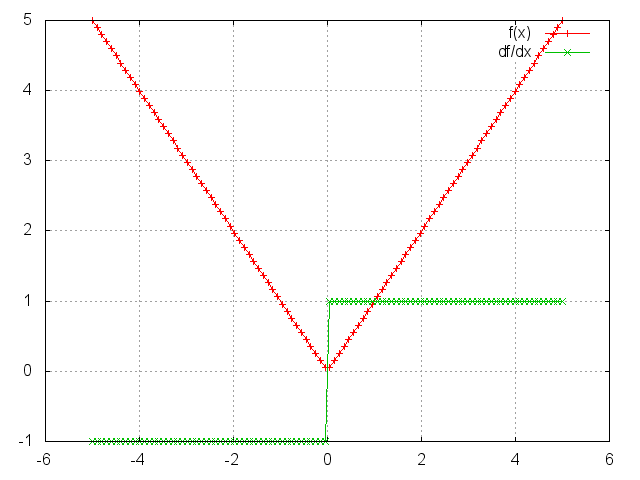`module` = `Add(inputDimension,scalar)`

Applies a bias term to the incoming data, i.e. y_i= x_i + b_i, or if _scalar=true then uses a single bias term, _y_i= x_i + b.

Example:

``````y=torch.Tensor(5);
mlp=nn.Sequential()

function gradUpdate(mlp, x, y, criterion, learningRate)
local pred = mlp:forward(x)
local err = criterion:forward(pred, y)
local gradCriterion = criterion:backward(pred, y)
mlp:updateParameters(learningRate)
return err
end

for i=1,10000 do
x=torch.rand(5)
y:copy(x);
for i=1,5 do y[i]=y[i]+i; end
end
print(mlp:get(1).bias)
``````

gives the output:

`````` 1.0000
2.0000
3.0000
4.0000
5.0000
[torch.Tensor of dimension 5]
``````

i.e. the network successfully learns the input x has been shifted to produce the output y.

## Mul

`module` = `Mul()`

Applies a single scaling factor to the incoming data, i.e. y= w x, where w is a scalar.

Example:

``````y=torch.Tensor(5);
mlp=nn.Sequential()

function gradUpdate(mlp, x, y, criterion, learningRate)
local pred = mlp:forward(x)
local err = criterion:forward(pred,y)
local gradCriterion = criterion:backward(pred,y);
mlp:updateParameters(learningRate);
return err
end

for i=1,10000 do
x=torch.rand(5)
y:copy(x); y:mul(math.pi);
end
print(mlp:get(1).weight)
``````

gives the output:

`````` 3.1416
[torch.Tensor of dimension 1]
``````

i.e. the network successfully learns the input `x` has been scaled by pi.

## CMul

`module` = `CMul(size)`

Applies a component-wise multiplication to the incoming data, i.e. `y_i = w_i * x_i`. Argument `size` can be one or many numbers (sizes) or a `torch.LongStorage`. For example, `nn.CMul(3,4,5)` is equivalent to `nn.CMul(torch.LongStorage{3,4,5})`.

Example:

``````mlp=nn.Sequential()

y=torch.Tensor(5);
sc=torch.Tensor(5); for i=1,5 do sc[i]=i; end -- scale input with this

local pred = mlp:forward(x)
local err = criterion:forward(pred,y)
local gradCriterion = criterion:backward(pred,y);
mlp:updateParameters(learningRate);
return err
end

for i=1,10000 do
x=torch.rand(5)
y:copy(x); y:cmul(sc);
end
print(mlp:get(1).weight)
``````

gives the output:

`````` 1.0000
2.0000
3.0000
4.0000
5.0000
[torch.Tensor of dimension 5]
``````

i.e. the network successfully learns the input x has been scaled by those scaling factors to produce the output y.

## Max

`module` = `Max(dimension)`

Applies a max operation over dimension `dimension`. Hence, if an `nxpxq` Tensor was given as input, and `dimension` = `2` then an `nxq` matrix would be output.

## Min

`module` = `Min(dimension)`

Applies a min operation over dimension `dimension`. Hence, if an `nxpxq` Tensor was given as input, and `dimension` = `2` then an `nxq` matrix would be output.

## Mean

`module` = `Mean(dimension)`

Applies a mean operation over dimension `dimension`. Hence, if an `nxpxq` Tensor was given as input, and `dimension` = `2` then an `nxq` matrix would be output.

## Sum

`module` = `Sum(dimension)`

Applies a sum operation over dimension `dimension`. Hence, if an `nxpxq` Tensor was given as input, and `dimension` = `2` then an `nxq` matrix would be output.

## Euclidean

`module` = `Euclidean(inputSize,outputSize)`

Outputs the Euclidean distance of the input to `outputSize` centers, i.e. this layer has the weights `w_j`, for `j` = `1`,..,`outputSize`, where `w_j` are vectors of dimension `inputSize`.

The distance `y_j` between center `j` and input `x` is formulated as `y_j = || w_j - x ||`.

## WeightedEuclidean

`module` = `WeightedEuclidean(inputSize,outputSize)`

This module is similar to Euclidean, but additionally learns a separate diagonal covariance matrix across the features of the input space for each center.

In other words, for each of the `outputSize` centers `w_j`, there is a diagonal covariance matrices `c_j`, for `j` = `1`,..,`outputSize`, where `c_j` are stored as vectors of size `inputSize`.

The distance `y_j` between center `j` and input `x` is formulated as `y_j = || c_j * (w_j - x) ||`.

## Identity

`module` = `Identity()`

Creates a module that returns whatever is input to it as output. This is useful when combined with the module ParallelTable in case you do not wish to do anything to one of the input Tensors. Example:

``````mlp=nn.Identity()
print(mlp:forward(torch.ones(5,2)))
``````

gives the output:

`````` 1  1
1  1
1  1
1  1
1  1
[torch.Tensor of dimension 5x2]
``````

Here is a more useful example, where one can implement a network which also computes a Criterion using this module:

``````pred_mlp=nn.Sequential(); -- A network that makes predictions given x.

xy_mlp=nn.ParallelTable();-- A network for predictions and for keeping the
xy_mlp:add(pred_mlp)      -- true label for comparison with a criterion
xy_mlp:add(nn.Identity()) -- by forwarding both x and y through the network.

mlp=nn.Sequential();     -- The main network that takes both x and y.
mlp:add(xy_mlp)      -- It feeds x and y to parallel networks;
cr=nn.MSECriterion();
cr_wrap=nn.CriterionTable(cr)
mlp:add(cr_wrap)         -- and then applies the criterion.

for i=1,100 do       -- Do a few training iterations
x=torch.ones(5);          -- Make input features.
y=torch.Tensor(3);
y:copy(x:narrow(1,1,3)) -- Make output label.
err=mlp:forward{x,y}    -- Forward both input and output.
print(err)         -- Print error from criterion.

mlp:zeroGradParameters();  -- Do backprop...
mlp:backward({x, y} );
mlp:updateParameters(0.05);
end
``````

## Copy

`module` = `Copy(inputType,outputType,[forceCopy,dontCast])`

This layer copies the input to output with type casting from input type from `inputType` to `outputType`. Unless `forceCopy` is true, when the first two arguments are the same, the input isn't copied, only transfered as the output. The default `forceCopy` is false. When `dontCast` is true, a call to `nn.Copy:type(type)` will not cast the module's `output` and `gradInput` Tensors to the new type. The default is false.

## Narrow

`module` = `Narrow(dimension, offset, length)`

Narrow is application of narrow operation in a module.

## Replicate

`module` = `Replicate(nFeature)`

This class creates an output where the input is replicated `nFeature` times along its first dimension. There is no memory allocation or memory copy in this module. It sets the stride along the first dimension to zero.

``````torch> x=torch.linspace(1,5,5)
torch> =x
1
2
3
4
5
[torch.DoubleTensor of dimension 5]

torch> m=nn.Replicate(3)
torch> o=m:forward(x)
torch> =o
1  2  3  4  5
1  2  3  4  5
1  2  3  4  5
[torch.DoubleTensor of dimension 3x5]

torch> x:fill(13)
torch> =x
13
13
13
13
13
[torch.DoubleTensor of dimension 5]

torch> =o
13  13  13  13  13
13  13  13  13  13
13  13  13  13  13
[torch.DoubleTensor of dimension 3x5]

``````

## Reshape

`module` = `Reshape(dimension1, dimension2, ... [, batchMode])`

Reshapes an `nxpxqx..` Tensor into a `dimension1xdimension2x...` Tensor, taking the elements column-wise.

The optional last argument `batchMode`, when `true` forces the first dimension of the input to be considered the batch dimension, and thus keep its size fixed. This is necessary when dealing with batch sizes of one. When `false`, it forces the entire input (including the first dimension) to be reshaped to the input size. Default `batchMode=nil`, which means that the module considers inputs with more elements than the produce of provided sizes, i.e. `dimension1xdimension2x...`, to be batches.

Example:

``````> x=torch.Tensor(4,4)
> for i=1,4 do
>  for j=1,4 do
>   x[i][j]=(i-1)*4+j;
>  end
> end
> print(x)

1   2   3   4
5   6   7   8
9  10  11  12
13  14  15  16
[torch.Tensor of dimension 4x4]

> print(nn.Reshape(2,8):forward(x))

1   9   2  10   3  11   4  12
5  13   6  14   7  15   8  16
[torch.Tensor of dimension 2x8]

> print(nn.Reshape(8,2):forward(x))

1   3
5   7
9  11
13  15
2   4
6   8
10  12
14  16
[torch.Tensor of dimension 8x2]

> print(nn.Reshape(16):forward(x))

1
5
9
13
2
6
10
14
3
7
11
15
4
8
12
16
[torch.Tensor of dimension 16]
``````

## View

`module` = `View(sizes)`

This module creates a new view of the input tensor using the `sizes` passed to the constructor. The parameter `sizes` can either be a `LongStorage` or numbers.

The method `setNumInputDims()` allows to specify the expected number of dimensions of the inputs of the modules. This makes it possible to use minibatch inputs when using a size -1 for one of the dimensions.

Example 1:

``````> x=torch.Tensor(4,4)
> for i=1,4 do
>  for j=1,4 do
>   x[i][j]=(i-1)*4+j;
>  end
> end
> print(x)

1   2   3   4
5   6   7   8
9  10  11  12
13  14  15  16
[torch.Tensor of dimension 4x4]

> print(nn.View(2,8):forward(x))

1   2   3   4   5   6   7   8
9  10  11  12  13  14  15  16
[torch.DoubleTensor of dimension 2x8]

> print(nn.View(torch.LongStorage{8,2}):forward(x))

1   2
3   4
5   6
7   8
9  10
11  12
13  14
15  16
[torch.DoubleTensor of dimension 8x2]

> print(nn.View(16):forward(x))

1
2
3
4
5
6
7
8
9
10
11
12
13
14
15
16
[torch.DoubleTensor of dimension 16]
``````

Example 2:

``````> input = torch.Tensor(2,3)
> minibatch = torch.Tensor(5,2,3)
> m = nn.View(-1):setNumInputDims(2)
> print(#m:forward(input))

6
[torch.LongStorage of size 2]

> print(#m:forward(minibatch))

5
6
[torch.LongStorage of size 2]

``````

## Select

Selects a dimension and index of a `nxpxqx..` Tensor.

Example:

``````mlp=nn.Sequential();

x=torch.randn(10,5)
print(x)
print(mlp:forward(x))
``````

gives the output:

`````` 0.9720 -0.0836  0.0831 -0.2059 -0.0871
0.8750 -2.0432 -0.1295 -2.3932  0.8168
0.0369  1.1633  0.6483  1.2862  0.6596
0.1667 -0.5704 -0.7303  0.3697 -2.2941
0.4794  2.0636  0.3502  0.3560 -0.5500
-0.1898 -1.1547  0.1145 -1.1399  0.1711
-1.5130  1.4445  0.2356 -0.5393 -0.6222
-0.6587  0.4314  1.1916 -1.4509  1.9400
0.2733  1.0911  0.7667  0.4002  0.1646
0.5804 -0.5333  1.1621  1.5683 -0.1978
[torch.Tensor of dimension 10x5]

0.0369
1.1633
0.6483
1.2862
0.6596
[torch.Tensor of dimension 5]
``````

This can be used in conjunction with Concat to emulate the behavior of Parallel, or to select various parts of an input Tensor to perform operations on. Here is a fairly complicated example:

``````
mlp=nn.Sequential();
c=nn.Concat(2)
for i=1,10 do
local t=nn.Sequential()
end

pred=mlp:forward(torch.randn(10,3))
print(pred)

for i=1,10000 do     -- Train for a few iterations
x=torch.randn(10,3);
y=torch.ones(2,10);
pred=mlp:forward(x)

criterion= nn.MSECriterion()
err=criterion:forward(pred,y)
mlp:updateParameters(0.01);
print(err)
end
``````

## Exp

Applies the `exp` function element-wise to the input Tensor, thus outputting a Tensor of the same dimension.

``````ii=torch.linspace(-2,2)
m=nn.Exp()
oo=m:forward(ii)
go=torch.ones(100)
gi=m:backward(ii,go)
gnuplot.plot({'f(x)',ii,oo,'+-'},{'df/dx',ii,gi,'+-'})
gnuplot.grid(true)
``````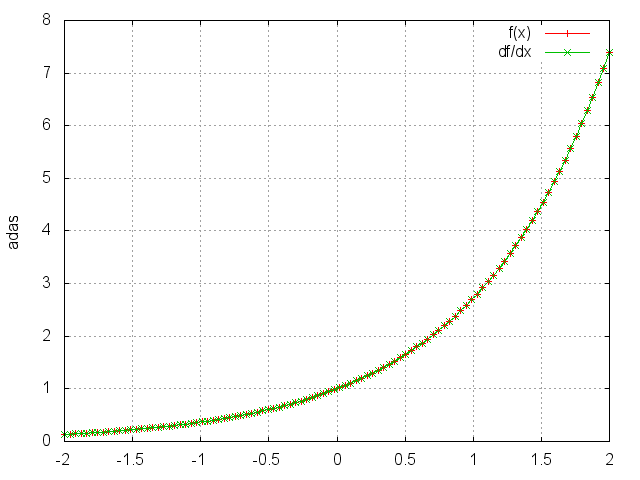## Square

Takes the square of each element.

``````ii=torch.linspace(-5,5)
m=nn.Square()
oo=m:forward(ii)
go=torch.ones(100)
gi=m:backward(ii,go)
gnuplot.plot({'f(x)',ii,oo,'+-'},{'df/dx',ii,gi,'+-'})
gnuplot.grid(true)
``````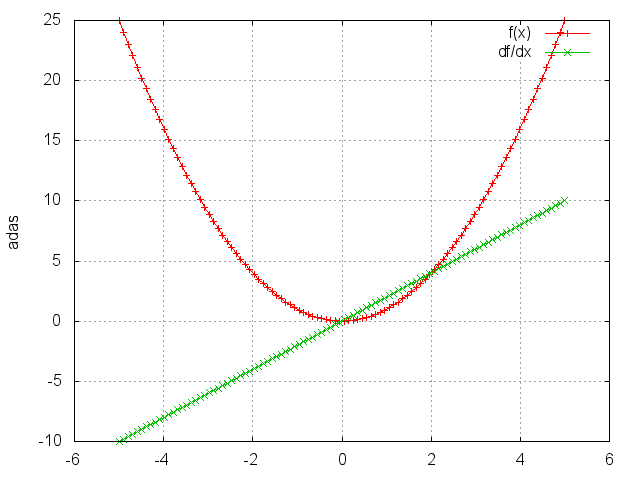## Sqrt

Takes the square root of each element.

``````ii=torch.linspace(0,5)
m=nn.Sqrt()
oo=m:forward(ii)
go=torch.ones(100)
gi=m:backward(ii,go)
gnuplot.plot({'f(x)',ii,oo,'+-'},{'df/dx',ii,gi,'+-'})
gnuplot.grid(true)
``````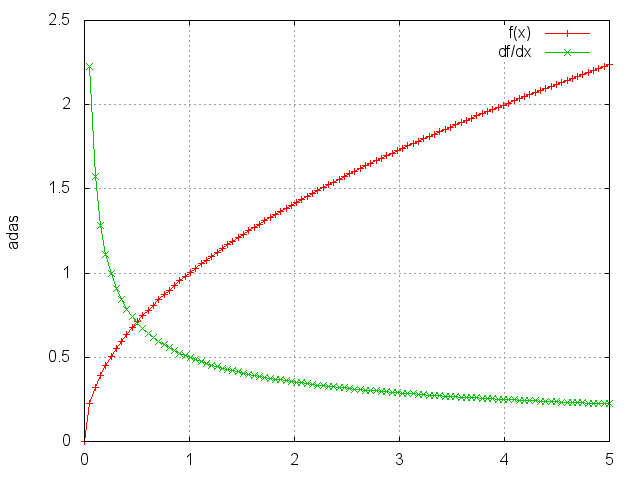## Power

`module` = `Power(p)`

Raises each element to its `pth` power.

``````ii=torch.linspace(0,2)
m=nn.Power(1.25)
oo=m:forward(ii)
go=torch.ones(100)
gi=m:backward(ii,go)
gnuplot.plot({'f(x)',ii,oo,'+-'},{'df/dx',ii,gi,'+-'})
gnuplot.grid(true)
``````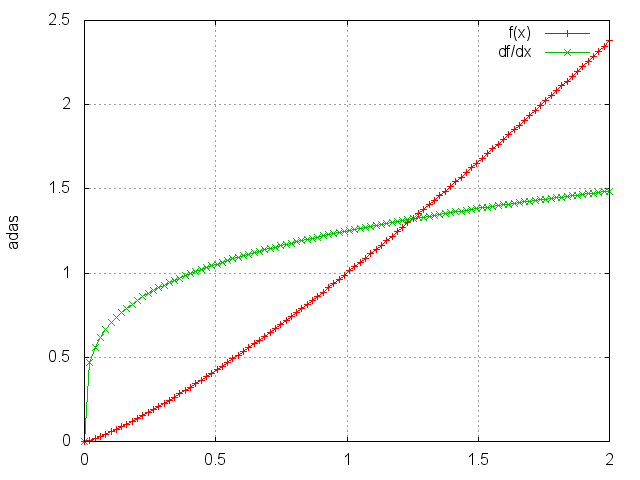## MM

`module` = `nn.MM(transA, transB)`

Performs multiplications on one or more pairs of matrices. If `transA` is set, the first matrix is transposed before multiplication. If `transB` is set, the second matrix is transposed before multiplication. By default, the matrices do not get transposed.

The module also accepts 3D inputs which are interpreted as batches of matrices. When using batches, the first input matrix should be of size `b x m x n` and the second input matrix should be of size `b x n x p` (assuming `transA` and `transB` are not set).

``````model = nn.MM()
A = torch.randn(b, m, n)
B = torch.randn(b, n, p)
C = model.forward({A, B})  -- C will be of size `b x m x n`
``````